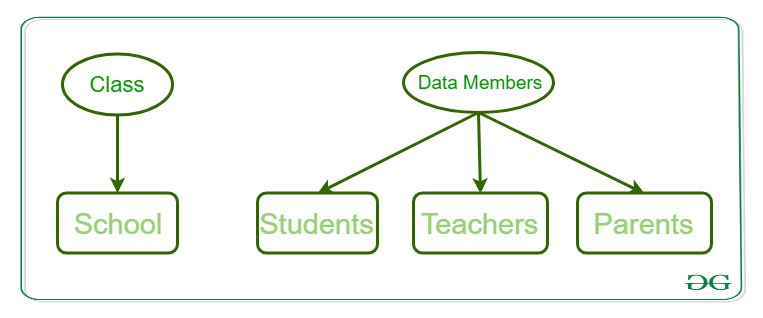Open in App
Not now

# Perl | Classes in OOP

• Last Updated : 11 Mar, 2022

In this modern world, where the use of programming has moved to its maximum and has its application in each and every work of our lives, we need to adapt ourselves to such programming paradigms that are directly linked to the real-world examples. There has been a drastic change in the competitiveness and the complexity of the real-world problems, thus the industry now requires some versatile approaches towards these complexities. The concept of Object-Oriented Programming language copes up comfortably with these complexities and is a perfect way of shaping up any programming language in a way that the real-world examples become very easy to solve. Object-Oriented Programming(OOP) uses the methodology of emphasizing more on objects rather than the procedure. The OOPs concept is about working in terms of objects and the interaction between objects.

Note: Object-oriented programming aims to implement real-world entities like inheritance, encapsulation, polymorphism, etc in programming. The main aim of OOP is to bind together the data and the functions that operate on them so that no other part of the code can access this data except that function.

## Object –

An object is an instance of a class of the data structure with some characteristics and behavior. For Example, we say an “Apple” is an object. Its characteristics are:it is a fruit, its color is red, etc. Its behavior is:”it tastes good“. In the concept of OOPs, the characteristics of an object are represented by its data and the behavior is represented by its associated functions. Thus an object is an entity that stores data and has its interface through functions.

## Class –

A class is an expanded concept of data structures. It defines a prototype blueprint of objects comprising of data. And an object is an instance of a class. A class comprises of the data members and data functions and it is a pre-defined data type made as per the user’s requirements which can be accessed and used by creating an instance of that class.

Example:
Consider a class of School. There may be schools with different names and structure but all of them share some common characteristics like students, teachers, staff, etc. So here school is the class with teachers, students, and Parents as the data members, and the member functions can be calculate_students_marks(), calculate_teachers_salary(), and Parents_Database().

Data Member: Data members are the data variables and member functions are the functions used to manipulate these variables and together these data members and member functions define the properties and behavior of the objects in a Class.### Defining a Class –

It is very easy to define a class in Perl. In Perl, a Class corresponds to a package. To define a class we first load and build a Package. A Package is a pre-contained unit of user-defined variables and subroutines, which can be used throughout the program.

Syntax:

`package Class_name`

### Creating Class and Using Objects

A class in Perl can be created by using the keyword package but to create an object, a constructor is called. A constructor is defined in a class as a method.

Creating an instance of a class
A class name and a constructor name can be as per user’s requirement. Most of the programmers prefer to use ‘new’ as a constructor name for their programs as it is easy to remember and is more feasible to use than any other complex constructor name.

## Perl

 `package` `student     // This is the class student` `sub` `Student_data    // Constructor to class` `{` `    ``my` `\$class` `= ``shift``;` `    ``my` `\$self` `= {` `                ``_StudentFirstName` `=> ``shift``;` `                ``_StudentLastName` `=> ``shift``;` `               ``};` `               `  `    ``print` `"Student's First Name is \$self ->{_StudentFirstName}\n"``;` `    ``print` `"Student's Last Name is \$self ->{_StudentLastName}\n"``;` `    ``bless` `\$self``, ``\$class``;` `    ``return` `\$self``;` `}`

In the above example code, a function named bless is used. This function is used to attach an object to a class which is passed to it as an argument.

Syntax:

`bless Object_name, Class Name`

Creating an Object
An object in Perl is instantiated by using the constructor defined in the class. An object name can be any variable as per user’s requirement, but it is conventional to name it with respect to the relevancy with the class.

## Perl

 `\$Data` `= Student_data student( ``"Shikhar"``, ``"Mathur"``);`

Example:

## Perl

 `use` `strict;` `use` `warnings;`   `package` `student;`   `# constructor` `sub` `student_data` `{`   `    ``# shift will take package name 'student' ` `    ``# and assign it to variable 'class'` `    ``my` `\$class_name` `= ``shift``;` `    ``my` `\$self` `= {` `                ``'StudentFirstName'` `=> ``shift``,` `                ``'StudentLastName'` `=> ``shift` `               ``};` `    ``# Using bless function` `    ``bless` `\$self``, ``\$class_name``;` `    `  `    ``# returning object from constructor` `    ``return` `\$self``;` `}`   `# Object creating and constructor calling` `my` `\$Data` `= new student_data student(``"Geeks"``,``"forGeeks"``);`   `# Printing the data` `print` `"\$Data->{'StudentFirstName'}\n"``;` `print` `"\$Data->{'StudentLastName'}\n"``;`

Output:

```Geeks
forGeeks```

The concept of using classes in Object-Oriented Programming is very important as it completely depicts the real-world applications and can be adopted in real-world problems.

My Personal Notes arrow_drop_up
Related Articles